This work focuses on the problem of perspective taking, which is the ability to take another agent’s point of view, either visually or conceptually.

For example, consider the scenario in Fig. 1 where a robot and a human are aligning a peg and hole to assemble a table. As the robot is holding the table, it cannot see the peg and hole. To collaborate effectively, the robot should reason about the human’s visual perspective and infer that the human is able to see the peg and hole (despite not knowing what he actually sees). It can then query the human for relevant information.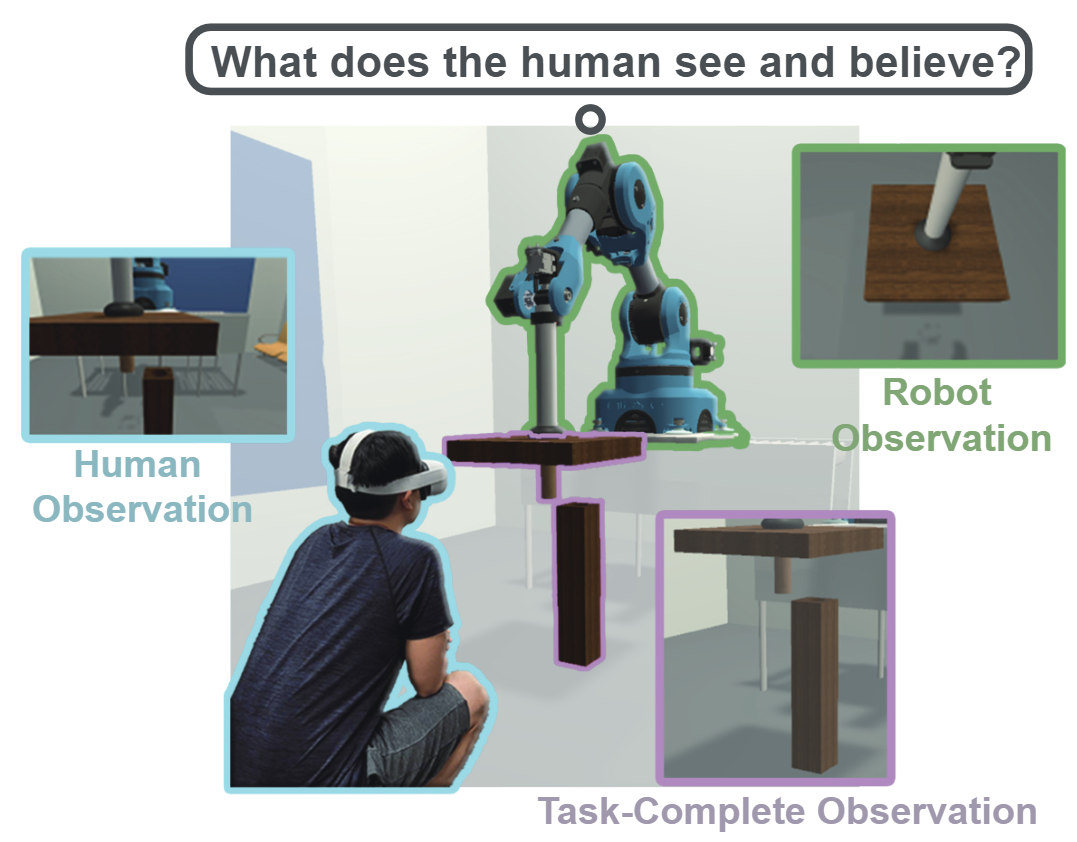Fig 1. An example of a table assembly task. The robot (green) is holding the table, it cannot see the peg and hole (purple), which is clearly visible to the human (light blue). The robot considers the perspective of the human and reasons about what the human can observe, despite not knowing what he actually sees.

However, enabling robots to perform perspective-taking remains an unsolved problem; existing approaches that use deterministic  or handcrafted methods  are unable to accurately account for uncertainty in partially-observable settings. We propose to address this limitation via a deep world model that enables a robot to perform both perception and conceptual perspective taking, i.e., the robot is able to infer what a human sees and believes.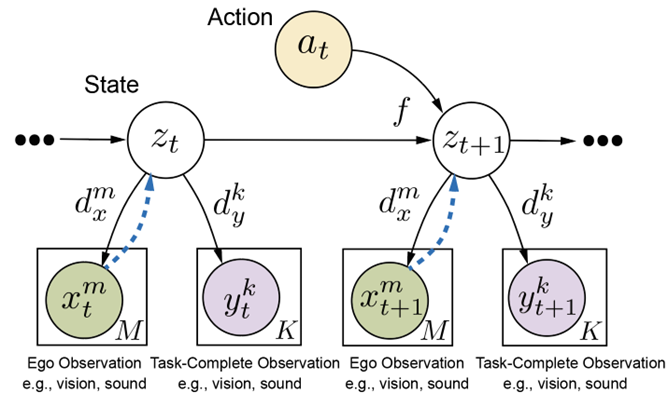Fig 2. Probabilistic graphic model for multimodal latent state-space model.

We start by a standard multimodal latent state-space model (MSSM) with a single time-dependent latent variable (Fig. 2). In general, the robot is unable to directly perceive the entire world state and we distinguish between two kinds of observations: 1) Ego observations $$x^m_t$$ at time $$t$$ for $$m = 1, \cdots, M$$ sensory modalities, which are the agent’s observations from its own perspective (e.g., a camera mounted on the robot’s arm) and can be accessed in both training and testing;

2) Task-Complete observations $$y^k_t$$ at time $$t$$ for $$k = 1, \cdots , K$$ modalities, which contains all sufficient information about world state to complete the task and can only be accessed during training.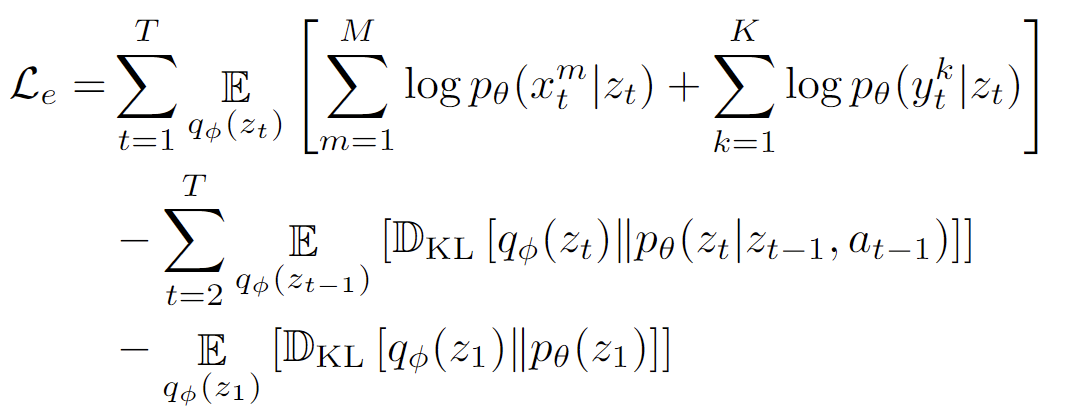The problem lies in using this inference network during test time when the task-complete observations are missing. One simple solution is to randomly drop the task-complete observations during training by zero-ing out $$y^{1:M}_{1:t}$$. Unfortunately, our preliminary experiments results showed that this approach led to poor variational distributions: $$q_\phi(z_t)$$ was ‘narrow’ (over-confident) when $$x^{1:M}_{1:t}$$ did not contain sufficient information of the world state.

One possible reason for this undesirable behavior is that the MSSM is trained to encode $$y^{1:M}_{t}$$ into $$z_t$$ via the reconstruction term $$\mathop{\mathbb{E}}_{q_{\phi}(z_{t})}\left[\log p_{\theta}(y^k_{t}|z_{t})\right]$$. If we drop $$y^{1:M}_{1:t}$$, q(zt|uφ(x1:M 1:t )) may lack sufficient information to generate a sample representative of the world state. In such cases, the model learns to “hedge” by generating (decoding) the mean observation, which corresponds to a narrow $$q_\phi(z_t)$$ centered around a specific latent state.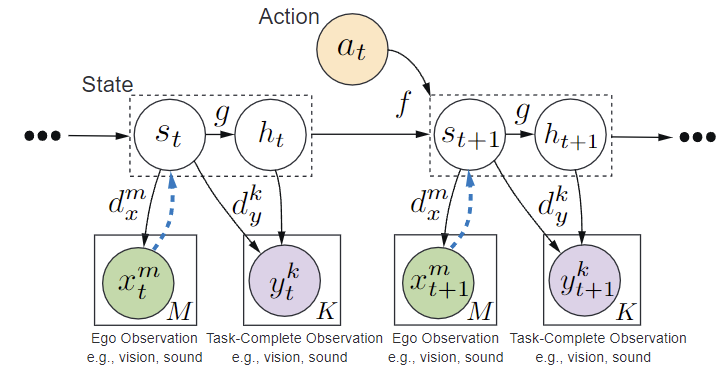Fig 3. Probabilistic graphic model for LEAPT.

As a remedy, we propose to restructure the MSSM to have a more explicit generation process. This modified structure is illustrated in Fig. 3. Intuitively, we would like the model to learn how to generate task-complete observations only using ego observations. As such, we decompose the latent state into two disjoint parts, $$z_t = [s_t, h_t]$$, where $$s_t$$ represents the observable part of the world and $$h_t$$ represents information that the robot cannot observe directly. By conditioning $$h_t$$ on $$s_t$$ in $$p(h_{t}| g_{\theta}([s, h ,a]_{t-1},s_{t}))$$, the model explicitly represents our desired computation using a neural network $$g_{\theta}$$.

From this graph structure, the ELBO can be written as: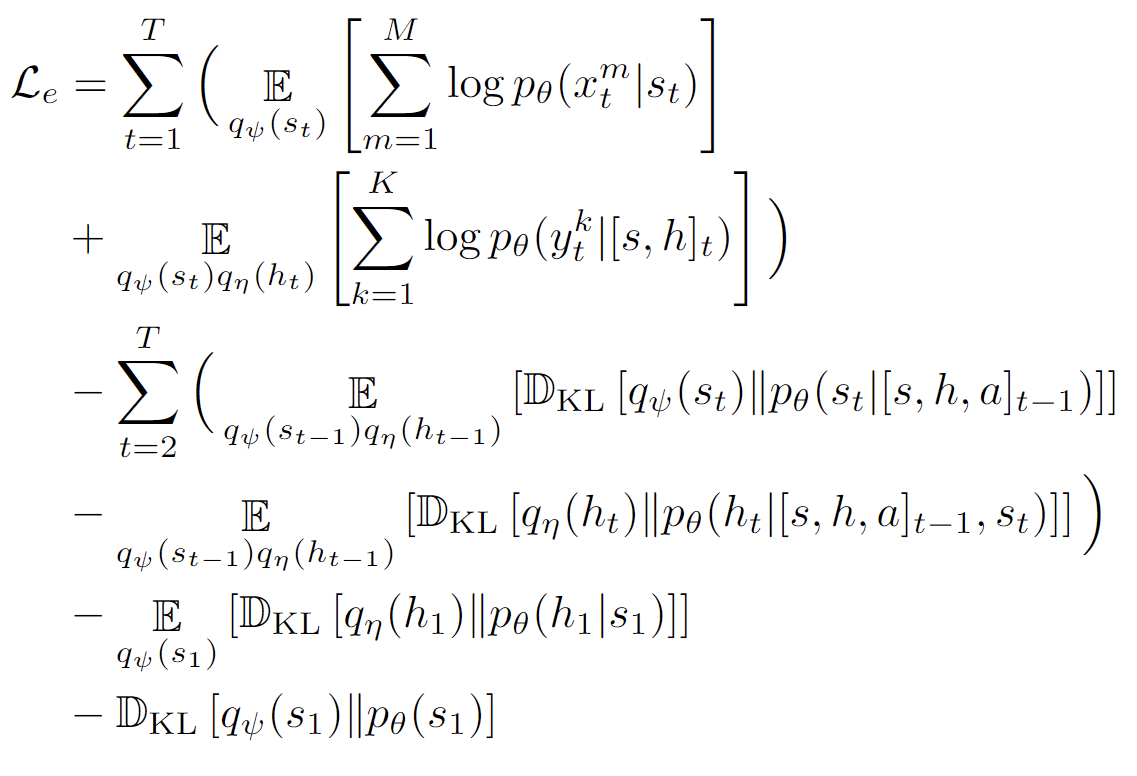In contrast to the MSSM, we don’t need to discard $$y^{1:K}_{1:t}$$ during the training process. Instead, we substitute $$q_{\eta}(h_t)$$ with $$p_{\theta}(h_t|[s,h, a]_{t-1}, s_{t})$$ during the testing phase (or with $$p_{\theta}(h_{1}|s_1)$$ when $$t=1$$). This ELBO variant enables $q_{\eta}(h_t)$ to convert $$y^{1:K}_{1:t}$$ into a latent distribution, such as a Gaussian or Categorical distribution. By minimizing $$\mathbb{D}_{\mathrm{KL}}\left[q_{\eta}(h_{t}) \| p_{\theta}(h_{t}|[s,h, a]_{t-1}, s_{t})\right]$$ and $$\mathbb{D}_{\mathrm{KL}}\left[q_{\eta}(h_{1}) \| p_{\theta}(h_{1}|s_1)\right]$$, $$p_{\theta}(h_{t}|[s,h, a]_{t-1}, s_{t})$$ and $$p_{\theta}(h_{1}|s_1)$$ are trained to generate potential $$h_t$$ using observations $$s_{1:t}$$. In a nutshell, this latent state-space model decomposition captures task-complete uncertainty at the latent level. By carefully setting $$q$$ and $$p$$, e.g., Gaussian, we can calculate these KL divergence terms exactly. We found that this decomposition resolves the issue of suboptimal latent state estimations and reconstructions, enabling us to create a self-model that produces credible samples.

We use sampled task-complete observations $$y^{1:K}_t$$ to generate relevant observations from different perspectives. Specifically, we train a visual perspective-taking model $$\hat{x}^{1:M}_{t} = d_\chi(y^{1:K}_{t}, \omega_t)$$, parameterized by $$\chi$$, that produces observations given an agent’s pose $$\omega_t$$ at time $$t$$ and $$y^{1:K}_t$$.

Training can be performed with data gathered by the robot $$\textrm{R}$$ during roll-outs in the environment. Given trajectories of the form $$\left\{(x^{1:M,\textrm{R}}_{t}, y^{1:K}_{t}, \omega_t^\textrm{R})\right\}_{t=1}^{T}$$ collected by the robot, we learn $$\chi$$ by minimizing the following loss:

$\mathcal{L}(\chi) = \sum_{t=1}^T \sum_{m=1}^M\left(\hat{x}^{m}_{t} - x^{m}_{t}\right)^2$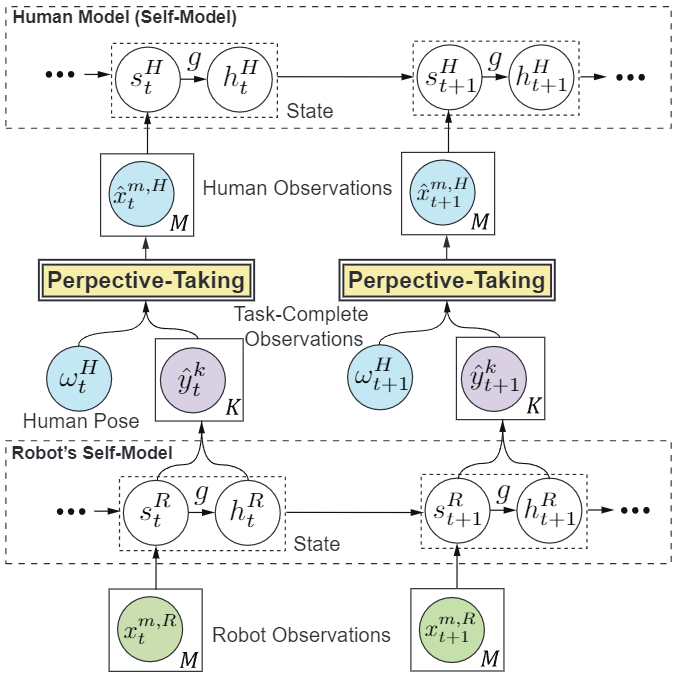Fig 4. Human belief inference. In brief, the robot’s world model (self-model) is used to sample possible environment states and predict human observations, and the human model is used to infer human belief. By leveraging the inference network and perspective taking-model, we can infer human belief under each sampled environment state.

Once trained, we can use the decomposed latent state space model and $$d_\chi$$ to obtain samples of another agent’s observations. The process is straightforward: given robot observations $$x^{1:M, \textrm{R}}_{t}$$ and the agent’s pose $$\omega_t^\textrm{H}$$, we sample a task-complete observation $$y^{1:K}_{t}$$ via (Fig. 4)

a) Sample the robot’s belief state $$s_t^\textrm{R} \sim q_\psi(s_t\|(\hat{x}^{1:M, \textrm{R}}_{t}))$$ and $$h_t^\textrm{R} \sim p_{\theta}(h_{t}\|[s^\textrm{R},h^\textrm{R}, a]_{t-1}, s^\textrm{R}_{t})$$ with $$h_1^\textrm{R} \sim p_{\theta}(h_{1}\|s^\textrm{R}_{1})$$.

b) Sample the task-complete observation $$\hat{y}^{1:K}_{t} \sim p_{\theta}(y^{1:K}_{t}\|s_t^\textrm{R}, h_t^\textrm{R})$$.

c) Given human position, generate human observations $$\hat{x}^{1:M, \textrm{H}}_{t}= d_\chi(\hat{y}^{1:K}_{t}, \omega_t^\textrm{H})$$

Next, we turn our attention to how the self-model can be used to estimate human belief, which is a form of conceptual perspective taking. We follow prior work  and use a variant of the robot’s self-model. By coupling the human model and robot’s self-model together as shown in Fig. 4., we sample belief states in the human model:

a) Generate the human observations $$\hat{x}^{1:M, \textrm{H}}_{1:t}$$ by visual perspective-taking.

b) Sample the human’s belief state $$s_t^\textrm{H}\sim q_\psi(s_t\|(\hat{x}^{1:M, \textrm{H}}_{1:t}))$$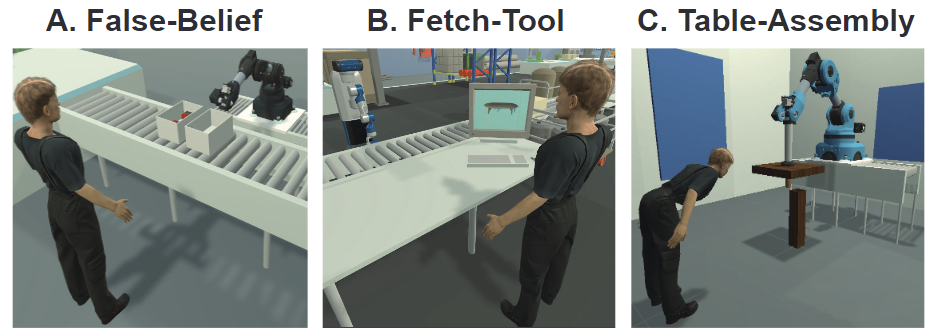Fig 5. Experimental domains. (A) A False-Belief test, where the robot cannot see what is inside the box but the human can. The human first observes the position of the drill (in the left or right box) and then walks away. Then, unobserved by the human, the robot will either switch the box or not. The robot’s task is to infer whether the human would believe that the objects remain in their original position. (B) In the Fetch-Tool task, a human is sitting in front of his workstation and observes a randomly chosen object on his computer screen. There are six different types of objects, which are characterized by their color and type. The robot’s task is to reason about human uncertainty and what he sees without observing the object, using only communication information from the human. However, communication of the object has three levels of ambiguity: type, colour and both the type and colour of the object. Once the robot figures out what the human sees, it can subsequently fetch the appropriate tool to assemble the object. (C) In the Table-Assembly, the robot’s task is to fit the peg of a table piece into a hole with guidance from the human. However, the hole may be occluded depending on the agent’s position. As the robot is holding on to the table, only the human is able to observe the relative distance between the peg and hole. The robot’s task is to reason whether the human can see the hole so that the robot can move the table to match it.

Experiments on 3 tasks in a simulated domain (Fig.) show that our model can better estimate the visual perceptions and beliefs of other agents compared to a deterministic approach and a standard latent-state space model. Specifically, we show our model enables robots to model false beliefs and better estimate another agent’s (and it’s own) beliefs during interaction and communication. We also report on a human-subject study using Virtual Reality (VR), which shows that LEAPT is better at predicting the human’s belief about the world.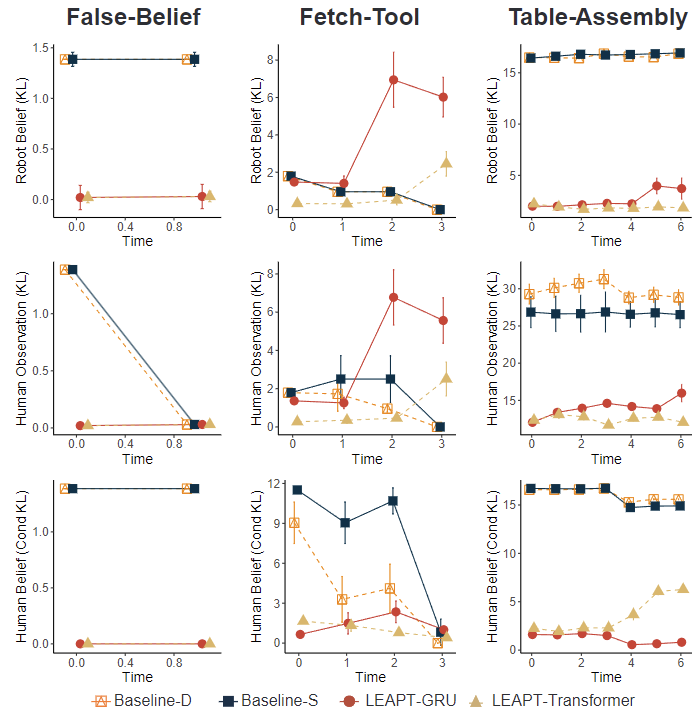Fig 6. Evaluation of (visual & conceptual) perspective-taking in three tasks using KL divergence as a measure (lower is better). In most cases, LEAPT-Transformer predicts the (simulated) human’s observation and belief more accurately compared to the other methods.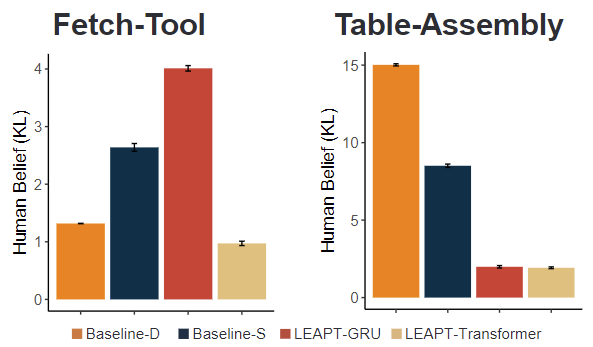Fig 7. Human-subject experiment results: KL divergence between predicted and actual human belief. The scores were averaged over the episode length. Overall, the LEAPT-Transformer achieves the best performance (lowest KL divergence).

## Code

Code for reproducing experiments presented in this paper can be found at this Github repo.

## Citation

If you find our code or the ideas presented in our paper useful for your research, consider citing our paper.

Kaiqi Chen, Jing Yu Lim, Kingsley Kuan, and Harold Soh. “Latent Emission-Augmented Perspective-Taking (LEAPT) for Human-Robot Interaction” International Conference on Robotics and Automation, 2023.

@article{chen2023latent,
title={Latent Emission-Augmented Perspective-Taking (LEAPT) for Human-Robot Interaction},
author={Chen, Kaiqi and Lim, Jing Yu and Kuan, Kingsley and Soh, Harold},
booktitle={2022 IEEE/RSJ International Conference on Intelligent Robots and Systems (IROS)},
year={2023}
}


## Acknowledgements

This work was supported by the Science and Engineering Research Council, Agency of Science, Technology and Research, Singapore, through the National Robotics Program under Grant No. 192 25 00054.

 B. Chen, Y. Hu, R. Kwiatkowski, S. Song, and H. Lipson, “Visual perspective taking for opponent behavior modeling,” in 2021 IEEE International Conference on Robotics and Automation (ICRA). IEEE, 2021, pp. 13 678–13 685.

 G. Milliez, M. Warnier, A. Clodic, and R. Alami, “A framework for endowing an interactive robot with reasoning capabilities about perspective-taking and belief management,” in The 23rd IEEE international symposium on robot and human interactive communication. IEEE, 2014, pp. 1103–1109.

 K. Chen, J. Fong, and H. Soh, “Mirror: Differentiable deep social projection for assistive human-robot communication,” in Proceedings of Robotics: Science and Systems, June 2022.

Written by